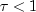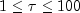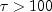JMSLTM Numerical Library 7.2.0
com.imsl.math

## Class Eigen

• ```public class Eigen
extends Object```
Collection of Eigen System functions.

`Eigen` computes the eigenvalues and eigenvectors of a real matrix. The matrix is first balanced. Orthogonal similarity transformations are used to reduce the balanced matrix to a real upper Hessenberg matrix. The implicit double-shifted QR algorithm is used to compute the eigenvalues and eigenvectors of this Hessenberg matrix. The eigenvectors are normalized such that each has Euclidean length of value one. The largest component is real and positive.

The balancing routine is based on the EISPACK routine `BALANC`. The reduction routine is based on the EISPACK routines `ORTHES` and `ORTRAN`. The QR algorithm routine is based on the EISPACK routine `HQR2`. See Smith et al. (1976) for the EISPACK routines. Further details, some timing data, and credits are given in Hanson et al. (1990).

While the exact value of the performance index,, is highly machine dependent, the performance of `Eigen` is considered excellent if, good if, and poor if.

The performance index was first developed by the EISPACK project at Argonne National Laboratory; see Smith et al. (1976, pages 124-125).

Example
• ### Nested Class Summary

Nested Classes
Modifier and Type Class and Description
`static class ` `Eigen.DidNotConvergeException`
The iteration did not converge
• ### Constructor Summary

Constructors
Constructor and Description
`Eigen()`
Constructs the eigenvalues and the eigenvectors of a real square matrix.
• ### Method Summary

Methods
Modifier and Type Method and Description
`int` `getMaxIterations()`
Returns the maximum number of iterations.
`Complex[]` `getValues()`
Returns the eigenvalues of a matrix of type `double`.
`Complex[][]` `getVectors()`
Returns the eigenvectors.
`double` `performanceIndex(double[][] a)`
Returns the performance index of a real eigensystem.
`void` `setMaxIterations(int maxIterations)`
Set the maximum number of iterations allowed.
`void` ```solve(double[][] a, boolean computeVectors)```
Solves for the eigenvalues and (optionally) the eigenvectors of a real square matrix.
• ### Methods inherited from class java.lang.Object

`clone, equals, finalize, getClass, hashCode, notify, notifyAll, toString, wait, wait, wait`
• ### Constructor Detail

• #### Eigen

`public Eigen()`
Constructs the eigenvalues and the eigenvectors of a real square matrix.
• ### Method Detail

• #### getMaxIterations

`public int getMaxIterations()`
Returns the maximum number of iterations.
Returns:
an `int` containing the maximum number of iterations.
• #### getValues

`public Complex[] getValues()`
Returns the eigenvalues of a matrix of type `double`.
Returns:
a `Complex` array containing the eigenvalues of this matrix in descending order
• #### getVectors

`public Complex[][] getVectors()`
Returns the eigenvectors.
Returns:
A `Complex` matrix containing the eigenvectors. The eigenvector corresponding to the j-th eigenvalue is stored in the j-th column. Each vector is normalized to have Euclidean length one.
• #### performanceIndex

`public double performanceIndex(double[][] a)`
Returns the performance index of a real eigensystem.
Parameters:
`a` - a `double` matrix
Returns:
A `double` scalar value indicating how well the algorithms which have computed the eigenvalue and eigenvector pairs have performed. A performance index less than 1 is considered excellent, 1 to 100 is good, while greater than 100 is considered poor.
• #### setMaxIterations

`public void setMaxIterations(int maxIterations)`
Set the maximum number of iterations allowed.
Parameters:
`maxIterations` - an `int` specifying the maximum number of iterations allowed. `maxIterations` must be greater than 0. By default, `maxIterations` = 50.
• #### solve

```public void solve(double[][] a,
boolean computeVectors)
throws Eigen.DidNotConvergeException```
Solves for the eigenvalues and (optionally) the eigenvectors of a real square matrix.
Parameters:
`a` - is the `double` square matrix for which the eigenvalues and (optionally) eigenvectors are to be found
`computeVectors` - is true if the eigenvectors are to be computed
Throws:
`Eigen.DidNotConvergeException` - is thrown when the algorithm fails to converge on the eigenvalues of the matrix.
JMSLTM Numerical Library 7.2.0